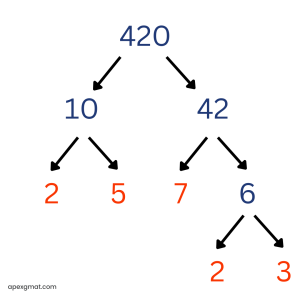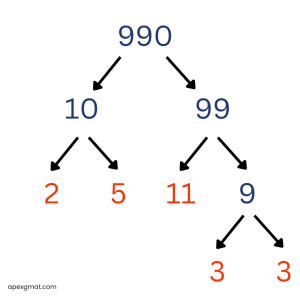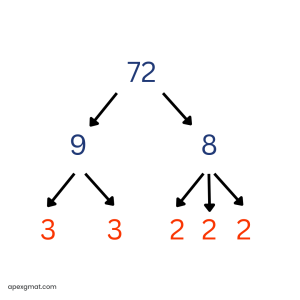Welcome back to our series on number properties. In this article, we cover the heart and soul of number properties: divisibility, specifically as it relates to the prime factors of integers. Here’s the all-important fact:

## Divisibility boils down to shared primes.

Given two integers n and x, n is divisible by x if and only if n contains every prime factor of x. Said another way, n is divisible by x if and only if every prime factor of x is also a prime factor of n. Prime factors thus serve as a “litmus test” for one integer’s divisibility by another. If n has all the prime factors of x, then n is divisible by x. If n does not have all the prime factors of x, then n is not divisible by x.

Since this is true, the best way to think of divisibility on GMAT quant is in terms of prime factors. If a question asks you whether n is divisible by x, you should translate that to the question, “Does n have all the prime factors of x?” This view of divisibility will help you through the problems built around the concept of divisibility.

Recall that the prime factorization of an integer doesn’t just list the prime numbers that are factors of the integer – it also reveals how many of each prime factor it would take to produce the integer via multiplication. Here’s an example:It would be overly simplistic to say that the prime factors of 420 are 2, 3, 5, and 7. Rather, we should say that the prime factorization of 420 is 22 * 3 * 5 * 7, since there are two prime factors of 2 “in” the integer 420.

In order for another integer to be divisible by 420, it must contain at least two prime factors of 2, along with at least one 3, at least one 5, and at least one 7.

Prime factors are powerful number properties tools, and it is not just the presence but the power of each prime factor (how many times it occurs) in a given integer that matters.

## Official GMAT problem for practice

Let’s try this out on some official GMAT problems:

If n is a positive integer and the product of all the integers from 1 to n, inclusive, is divisible by 990, what is the least possible value of n?

(A) 8

(B) 9

(C) 10

(D) 11

(E) 12

First, we need to get our heads around the phrase, “the product of all the integers from 1 to n, inclusive.” We could translate this to algebra like so:

1 * 2 * 3 * . . . *n

But there is a ready-made piece of notation for representing this concept that many GMAT problems intentionally ignore. Instead of “the product of all the integers from 1 to n, inclusive,” they could have simply said: n!

The exclamation point is called a factorial and represents the reversal of what this question verbally described: the product of all the integers from n to 1, inclusive. Here’s an example:

n = 6

n! = 6 * 5 * 4 * 3 * 2 * 1

Since the final factor of 1 does not affect the value of the expression, it is often omitted from “spelled out” forms of factorials that list the series of factors.

Now to handle the question being asked: if n! is divisible by 990, what is the least possible value of n? How low can n go?

Since divisibility is a matter of prime factors, the best way to proceed is to perform a prime factorization of 990. To correctly answer this question, we must select the lowest value of n such that n! contains all the prime factors of 990.990 = 2 * 32 * 5 * 11

The prime factor of 11 controls this problem. Here’s a helpful fact to remember about factorials and prime factors: a factorial will never contain a prime factor greater than its own “leading factor.” I use the term “leading factor” for the integer displayed with the exclamation point. When the factorial is “spelled out,” this integer always appears first.

To apply this fact, 10! does not contain a prime factor of 11. Since 11 is prime, it can only be produced by the multiplication 1 * 11. This is what it means to be prime. So there are no factors hiding within 10! that can be cleverly multiplied together to produce an 11. Feel free to try. The lowest factorial that contains a prime factor of 11 is 11! Therefore, in order for n! to be divisible by 990, n can be no lower than 11 – the highest prime factor of 990. The correct answer to this problem is D.

### Divisibility problem

Here’s another official problem:

A school administrator will assign each student in a group of n students to one of m classrooms. If 3 < m < 13 < n, is it possible to assign each of the n students to one of the m classrooms so that each classroom has the same number of students assigned to it?

1. It is possible to assign each of 3n students to one of m classrooms so that each classroom has the same number of students assigned to it.
2. It is possible to assign each of 13n students to one of m classrooms so that each classroom has the same number of students assigned to it.

(A) Statement (1) alone is sufficient, but statement (2) alone is not sufficient.

(B) Statement (2) alone is sufficient, but statement (1) alone is not sufficient.

(C) BOTH statements TOGETHER are sufficient, but NEITHER statement ALONE is sufficient.

(D) EACH statement ALONE is sufficient.

(E) Statements (1) and (2) TOGETHER are not sufficient.

This is a very wordy way to ask a very simple question: is n divisible by m? Here are some steps for translating this problem down to the simple concept of divisibility:

→ Is it possible to assign each of the n students to one of the m classrooms so that each classroom has the same number of students assigned to it?

→ Can n students be evenly divided into m classrooms?

→ Is n divisible by m?

→ Does n contain all the prime factors of m?

The final step of stating this question in terms of prime factors is necessary. Let’s translate the two statements as well.

1. 3n is divisible by m. → 3n contains all the prime factors of m.
2. 13n is divisible by m. → 13n contains all the prime factors of m.

### Terms of prime factors: Difference between the qualitatively-similar statements

When we put these statements in terms of prime factors, the key difference between the qualitatively-similar statements appears: the 13 in statement 2 is prime, while the 3 in statement 1 is not.

Recall from the question stem that m, the number of classrooms, is greater than 3 and less than 13. In terms of prime factors, this means that m could have a prime factor of 3 (if m equals 6 or 9 or 12), but it cannot have a prime factor of 13. As in the previous factorial problem, none of the factors of m can be cleverly multiplied together to produce a 13.

Let’s think about what this means for the sufficiency of statement 2. If m does not contain a prime factor of 13, and if 13n contains all the prime factors of m (this is what the statement tells us), then all of the prime factors of m are contained within n: n’s coefficient of 13 is not a prime factor of m and is therefore not contributing to the divisibility. It is “dead weight.” So from statement 2, n is divisible by m. Sufficient.

Statement 1 is different, because 3 might be a prime factor of m but not of n. If this is true, then n is not divisible by m, while 3n might be. Statement 1 basically says, “If we throw in an extra prime factor of 3 to the integer n, then it’s divisible by m.” But this extra prime factor of 3 may be the only thing making n divisible by m. We can’t know whether n alone, without the help of the 3 coefficient, is still divisible by m. Again, this is because 3 could be a prime factor of m. In statement 2, 13 cannot be a prime factor of m, so it cannot be “helping” n to be divisible by m.

Statement 2 is sufficient, but statement 1 is not. The correct answer is B.

### Factor/Divisor of n problem

Here’s a final (easier) problem to end the article:

If n is a positive integer and n2 is divisible by 72, then the largest positive integer that must divide n is

(A) 6

(B) 12

(C) 24

(D) 36

(E) 48

We are looking for the largest integer that is a factor/divisor of n, that is, the largest integer whose prime factors are all contained within n. Let’s translate what we know into prime factor data. “n2 is divisible by 72” means that n2 has all the prime factors of 72.72 = 23 * 32

Since n2 is divisible by 72, n2 has at least three prime factors of 2 and at least two prime factors of 3. To use this information, we need to remember another fact about the prime factors of perfect squares: perfect squares always have prime factors in pairs, or to put it another way, an even number of each prime factor. Since n2 is a perfect square, it cannot have exactly three prime factors of 2: it must have at least four prime factors of 2.

When the prime factors of a perfect square are split into two identical groups, each group represents the prime factors of the square root. If we split the known prime factors of n2, into two identical groups, each group has two prime factors of 2 and one prime factor of 3.

n2 = 2 * 2 * 2 * 2 * 3 * 3

n = 2 * 2 * 3

n2 and n could have more prime factors than these, but these are the only factors we can be sure of from the given fact that n2 is divisible by 72. So the largest integer by which n is sure to be divisible is 2 * 2 * 3 = 12. The correct answer is B.

With an understanding of divisibility in terms of prime factors, you will be prepared for many GMAT number properties problems. In the next article, we’ll consider some logical rules related to factors and divisibility.

If you are looking for extra help in preparing for the GMAT, we offer extensive one-on-one GMAT tutoring. You can schedule a complimentary 30-minute consultation call with one of our tutors to learn more!

Contributor: Elijah Mize (Apex GMAT Instructor)